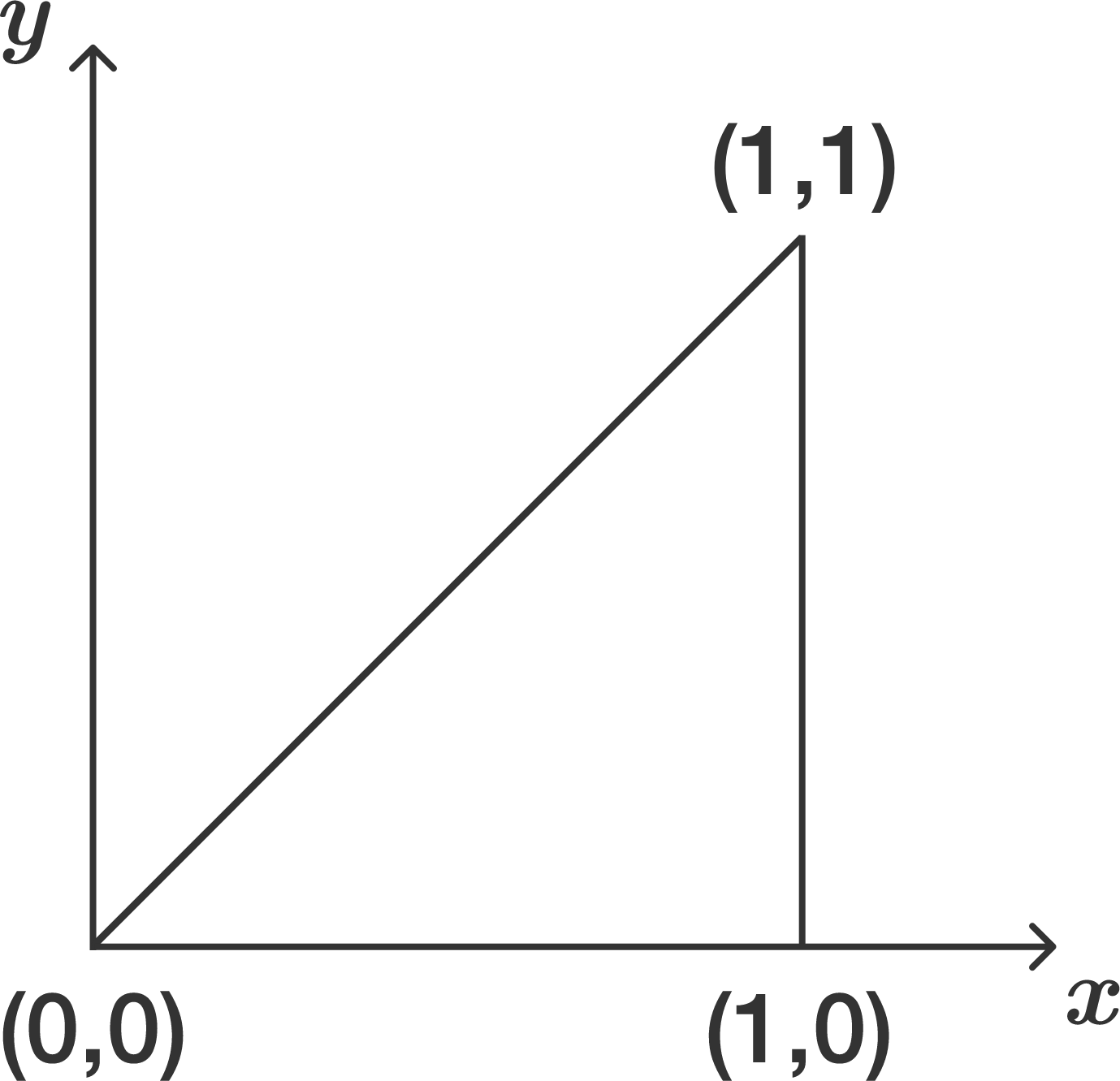# Voltage through space and timeIn the $xy$-plane, there is a triangular loop of conducting wire with vertices at $(0\text{ m},0\text{ m}), (1\text{ m},0\text{ m}),$ and $(1\text{ m},1\text{ m})$. There is a magnetic flux density oriented perpendicular to the $xy$-plane which is described by the equation below: $B = B_0 \sin\big(x^{2}+t\big),$ where the parameter $t$ denotes time and $B_0 = \SI[per-mode=symbol]{1}{\weber\per\meter\squared}$.

What is the magnitude (in volts) of the maximum voltage induced in the loop (to 3 decimal places)?

×